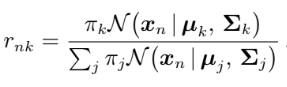# Gaussian Mixture Models: implemented from scratch

From the rising of the Machine Learning and Artificial Intelligence fields Probability Theory was a powerful tool, that allowed us to handle uncertainty in a lot of applications, from classification to forecasting tasks. Today I would like to talk with you more about the use of Probability and Gaussian distribution in clustering problems, implementing on the way the GMM model. So let’s get started

## What is GMM?

GMM (or Gaussian Mixture Models) is an algorithm that using the estimation of the density of the dataset to split the dataset in a preliminary defined number of clusters. For a better understandability, I will explain in parallel the theory and will show the code for implementing it.

For this implementation, I will use the EM (Expectation-Maximization) algorithm.

## The theory and the code is the best combination.

Firstly let’s import all needed libraries:

``````import numpy as np
import pandas as pd
``````

I highly recommend following the standards of sci-kit learn library when implementing a model on your own. That’s why we will implement GMM as a class. Let’s also the __init__ function.

``````class GMM:
def __init__(self, n_components, max_iter = 100, comp_names=None):
self.n_componets = n_components
self.max_iter = max_iter
if comp_names == None:
self.comp_names = [f"comp{index}" for index in range(self.n_componets)]
else:
self.comp_names = comp_names
# pi list contains the fraction of the dataset for every cluster
self.pi = [1/self.n_componets for comp in range(self.n_componets)]
``````

Shortly saying, n_components is the number of cluster in which whe want to split our data. max_iter represents the number of interations taken by the algorithm and comp_names is a list of string with n_components number of elements, that are interpreted as names of clusters.

## The fit function.

So before we get to the EM-algorithm we must split our dataset. after that, we must initiate 2 lists. One list containing the mean vectors (each element of the vector is the mean of columns) for every subset. The second list is containing the covariance matrix of each subset.

``````def fit(self, X):
# Spliting the data in n_componets sub-sets
new_X = np.array_split(X, self.n_componets)
# Initial computation of the mean-vector and covarience matrix
self.mean_vector = [np.mean(x, axis=0) for x in new_X]
self.covariance_matrixes = [np.cov(x.T) for x in new_X]
# Deleting the new_X matrix because we will not need it anymore
del new_X
``````

Now we can get to EM-algorithm.

## EM-algorithm.

As the name says the EM-algorithm is divided in 2 steps — E and M.

## E-step:

During the Estimation step, we calculate the r matrix. It is calculated using the formula below.The formula for calucalting the r (resposnsabilities) matrix (source — Mathemathis for Machine Learning Book)

r matrix is also known as ‘responsibilities’ and can be interpreted in the following way. Rows are the samples from the dataset, while columns represent every cluster, the elements of this matrix are interpreted as follows rnk is the probability of sample n to be part of cluster k. When the algorithm will converge we will use this matrix to predict the points cluster.

Also, we calculate the N list, in which each element is basically the sum of the correspondent column in the r matrix. The following code is doing that.

``````for iteration in range(self.max_iter):
''' ----------------   E - STEP   ------------------ '''
# Initiating the r matrix, evrey row contains the probabilities
# for every cluster for this row
self.r = np.zeros((len(X), self.n_componets))
# Calculating the r matrix
for n in range(len(X)):
for k in range(self.n_componets):
self.r[n][k] = self.pi[k] * self.multivariate_normal(X[n], self.mean_vector[k], self.covariance_matrixes[k])
self.r[n][k] /= sum([self.pi[j]*self.multivariate_normal(X[n], self.mean_vector[j], self.covariance_matrixes[j]) for j in range(self.n_componets)])
# Calculating the N
N = np.sum(self.r, axis=0)
``````

Point that the multivariate_normal is just the formular for normal distribution applyed to vectors, it is used to calculate the probability for vectors in a normal sitribution.

and the code bellow implement it, taking the row vector, mean vector and the covariance matrix.

``````def multivariate_normal(self, X, mean_vector, covariance_matrix):
return (2*np.pi)**(-len(X)/2)*np.linalg.det(covariance_matrix)**(-1/2)*np.exp(-np.dot(np.dot((X-mean_vector).T, np.linalg.inv(covariance_matrix)), (X-mean_vector))/2)
``````

Looks a little bit messy but, you can find the full code there.

## M-step:

During the Maximization-step we will step-by-step set the value fo the mean vectors and covariance matrices to describe with them the clusters. To do that we will use the following formulas.

In the code I will like that:

``````''' ---------------   M - STEP   --------------- '''
# Initializing the mean vector as a zero vector
self.mean_vector = np.zeros((self.n_componets, len(X)))
# Updating the mean vector
for k in range(self.n_componets):
for n in range(len(X)):
self.mean_vector[k] += self.r[n][k] * X[n]
self.mean_vector = [1/N[k]*self.mean_vector[k] for k in range(self.n_componets)]
# Initiating the list of the covariance matrixes
self.covariance_matrixes = [np.zeros((len(X), len(X))) for k in range(self.n_componets)]
# Updating the covariance matrices
for k in range(self.n_componets):
self.covariance_matrixes[k] = np.cov(X.T, aweights=(self.r[:, k]), ddof=0)
self.covariance_matrixes = [1/N[k]*self.covariance_matrixes[k] for k in range(self.n_componets)]
# Updating the pi list
self.pi = [N[k]/len(X) for k in range(self.n_componets)]
``````

And we are done with fit function. Etiratively applying EM-algorithm will make the GMM fianlly to converge.

## The predict function.

The predict function is actually very simple we simply use the multivariate normal function using the optimal mean vectors and covariance matrices for each cluster, to find using which gives the biggest values.

``````def predict(self, X):
probas = []
for n in range(len(X)):
probas.append([self.multivariate_normal(X[n], self.mean_vector[k], self.covariance_matrixes[k])
for k in range(self.n_componets)])
cluster = []
for proba in probas:
cluster.append(self.comp_names[proba.index(max(proba))])
return cluster
``````

## The result.

To test the model I chose to compare it with the GMM implemented in the sci-kit library. I generated 2 datasets using sci-kit learn dataset generating function — make_blobs with different setings. So that is the result.